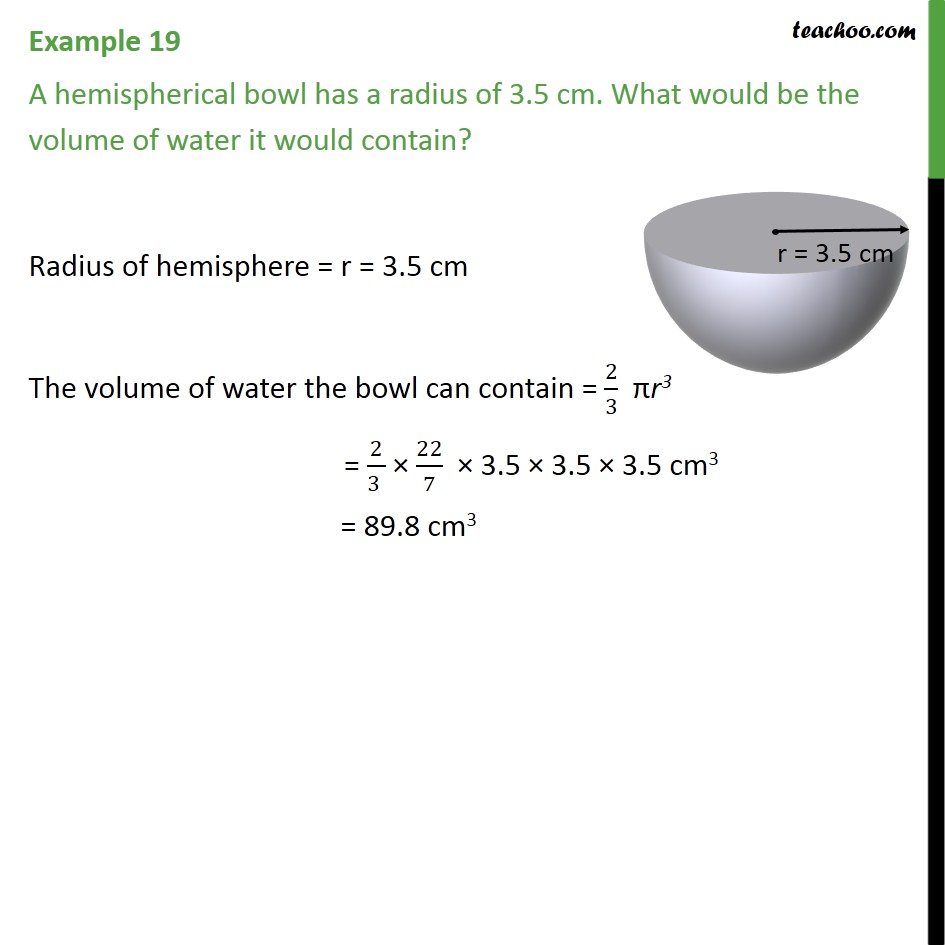Examples

Chapter 11 Class 9 Surface Areas and Volumes
Serial order wiseLearn in your speed, with individual attention - Teachoo Maths 1-on-1 Class

### Transcript

Example 12 A hemispherical bowl has a radius of 3.5 cm. What would be the volume of water it would contain? Radius of hemisphere = r = 3.5 cm The volume of water the bowl can contain = 2/3 πr3 = 2/(3 ) × 22/7 × 3.5 × 3.5 × 3.5 cm3 = 89.8 cm3